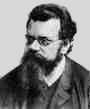Home

PHY 418: Statistical Mechanics I
Prof. S. Teitel stte@pas.rochester.edu ---- Spring 2012

## Problem Set 5

Due Wednesday, April 11, in lecture

• Problem 1 [10 points total]

Consider photons of a given energy ε = hbar ω.

(a) If <n> is the average number of such photons in equilibrium at temperature T, show that the fluctuation in the number of photons is

<n2> - <n>2 = - (1/ε) (d<n>/dβ)    where β = 1/kBT
[5 pts]

(b) Using the forumula for the equilibrium value of <n>, apply the above result to determine the relative fluctuation in the number of photons

[<n2> - <n>2]/<n>2
Is this large or small? [5 pts]

• Problem 2 [5 points]

The Stefan-Boltzmann law states that a black body at temperature T radiates power per unit surface area equal to σT4 where σ is a universal constant independent of the material properties of the body. Assuming that the sun and the earth are black bodies, and that the earth is in thermal equilibrium with the sun [i.e. energy absorbed = energy emitted] calclate the temperature of the earth in terms of the temperature of the sun. Look up the parameters you need in order to compute a number for this estimate of the earth's temperature.

• Problem 3 [30 points]

In the grand canonical ensemble, the probability to have a given state "a" with total energy Ea and total number of particles Na is ("a" is labeling the full N-particle state),

Pa = [e-(Ea-µNa)/kBT]/L
where
L = ∑a [e-(Ea-µNa)/kBT]       is the grand canonical partition function.

(a) For a quantum ideal gas, with single particle states i of energy εi, many particle states are specified by the occupation numbers {ni} and have energy E = ∑iini]. Show that the probability for the state with occupations {ni} is given by

P({ni}) = ∏i [pi(ni)]
where pi(ni) is the probability that single particle state i has occupation ni, and pi(ni) is given by
pi(ni) = [e-(εi-µ)ni/kBT]/wi
where
wi = ∑ni [e-(εi-µ)ni/kBT]
can be thought of as the partition function for the single particle state i. The above factorization says that the number of particles ni in state i, is independent of the number of particles nj in state j. [10 points]

(b) Using the above result, show that the Shannon definition of entropy can be written as

S = -kB{ni} [P({ni}) ln P({ni})] = -kBini [pi(ni) ln pi(ni)]
[10 points]

(c) Using the above result, show that the following expressions apply for the entropies of an ideal gas of bosons and fermions, respectively

 bosons: S = kB ∑i [(1+) ln (1+) - ln ] fermions: S = kB ∑i [-(1-) ln (1-) - ln ]
where <ni> = ∑}ni [ni pi(ni)] is the average occupation number of state i. [10 points]

• Problem 4 [15 points]

Consider a degenerate Fermi gas of non-interacting, non-relativisitic, particles in two dimensions (this might be a model for electrons in a thin metallic film).

a) Find the density of states g(ε).

b) Find the Fermi energy and the T=0 energy density.

c) Using the the fact that the particle density n is given by

 n = ∫ dε g(ε)eβ(ε-µ)+1
find the chemical potential as a function of temperature, µ(T), for fixed density n, by doing this integral exactly. You may have to look up an integral in an integral handbook! Using the exact expression for µ(T), find a simpler approximation that holds at low T<<TF. Does µ(T) have a power series expansion in T at low T, like we found in three dimensions with the Sommerfeld expansion?